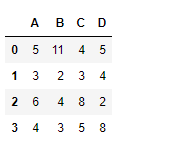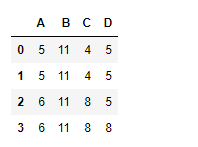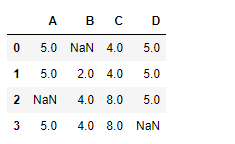# Python | Pandas dataframe.cummax()

Python is a great language for doing data analysis, primarily because of the fantastic ecosystem of data-centric python packages. Pandas is one of those packages and makes importing and analyzing data much easier.

Pandas` dataframe.cummax()` is used to find the cumulative maximum value over any axis. Each cell is populated with the maximum value seen so far.

Syntax: DataFrame.cummax(axis=None, skipna=True, *args, **kwargs)

Parameters:
axis : {index (0), columns (1)}
skipna : Exclude NA/null values. If an entire row/column is NA, the result will be NA

Returns: cummax : Series

Example #1: Use `cummax()` function to find the cumulative maximum value along the index axis.

 `# importing pandas as pd ` `import` `pandas as pd ` ` `  `# Creating the dataframe ` `df ``=` `pd.DataFrame({``"A"``:[``5``, ``3``, ``6``, ``4``], ` `                   ``"B"``:[``11``, ``2``, ``4``, ``3``], ` `                   ``"C"``:[``4``, ``3``, ``8``, ``5``],  ` `                   ``"D"``:[``5``, ``4``, ``2``, ``8``]}) ` ` `  `# Print the dataframe ` `df `

Output :Now find the cumulative maximum value over the index axis

 `# To find the cumulative max ` `df.cummax(axis ``=` `0``) `

Output :Example #2: Use `cummax()` function to find the cumulative maximum value along the column axis.

 `# importing pandas as pd ` `import` `pandas as pd ` ` `  `# Creating the dataframe ` `df ``=` `pd.DataFrame({``"A"``:[``5``, ``3``, ``6``, ``4``], ` `                   ``"B"``:[``11``, ``2``, ``4``, ``3``], ` `                   ``"C"``:[``4``, ``3``, ``8``, ``5``],  ` `                   ``"D"``:[``5``, ``4``, ``2``, ``8``]}) ` ` `  `# To find the cumulative max along column axis ` `df.cummax(axis ``=` `1``) `

Output :Example #3: Use `cummax()` function to find the cumulative maximum value along the index axis in a data frame with `NaN` value.

 `# importing pandas as pd ` `import` `pandas as pd ` ` `  `# Creating the dataframe ` `df ``=` `pd.DataFrame({``"A"``:[``5``, ``3``, ``None``, ``4``], ` `                   ``"B"``:[``None``, ``2``, ``4``, ``3``], ` `                   ``"C"``:[``4``, ``3``, ``8``, ``5``],  ` `                   ``"D"``:[``5``, ``4``, ``2``, ``None``]}) ` ` `  `# To find the cumulative max ` `df.cummax(axis ``=` `0``, skipna ``=` `True``) `

Output :My Personal Notes arrow_drop_upCheck out this Author's contributed articles.

If you like GeeksforGeeks and would like to contribute, you can also write an article using contribute.geeksforgeeks.org or mail your article to contribute@geeksforgeeks.org. See your article appearing on the GeeksforGeeks main page and help other Geeks.

Please Improve this article if you find anything incorrect by clicking on the "Improve Article" button below.

Article Tags :

Be the First to upvote.

Please write to us at contribute@geeksforgeeks.org to report any issue with the above content.